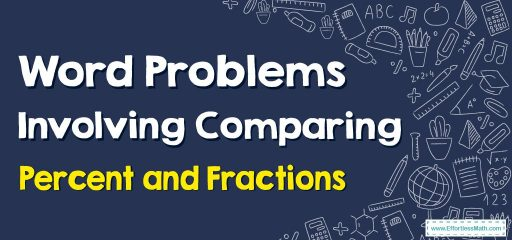# How to Solve Word Problems Involving Comparing Percent and Fractions?

In this step-by-step guide, you will learn how to solve different types of word problems involving comparing percentages and fractions.## A step-by-step guide to finding word problems involving comparing percent and fractions

Solving word problems involving comparisons between percentages and fractions can be challenging, but it can be done by following these steps:

1. Read the problem carefully and identify the information given. Make sure you understand what is being asked in the problem.
2. Identify the keywords or phrases that indicate a percentage or a fraction is involved. Words such as “percent,” “percentage,” “fraction,” “part,” or “whole” are clues.
3. Write an equation or a fraction to represent the information given in the problem. For example, if the problem states that $$40%$$ of a number is equal to $$\frac{3}{8}$$ of the same number, the equation would be $$0.40x = \frac{3}{8}x$$, where $$x$$ is the whole number.
4. Compare the given percentage and fraction by cross-multiplying and simplifying the equation. In this case, we have $$0.40x = \frac{3}{8}x$$. Cross-multiply and simplify we have: $$0.40 = \frac{3}{20}$$
5. Make a decision if the comparison is true or false, in this case, is false.
6. Provide the final answer in the context of the problem and make sure the units are clear and correct.

It’s important to remember that, when solving word problems involving comparisons between percentages and fractions, the key is to carefully read the problem, identify the information given, and translate that information into an equation or a fraction. Practice and understanding the keywords used in these problems will help you to solve them more efficiently.

### Word Problems Involving Comparing Percent and Fractions – Example 1

Yesterday Jacob drove 55% of the way to the next state. Today he drives $$\frac{2}{5}$$ of the way. Which day did he drive the most?
Solution:
Step 1:
Write a fraction as a decimal. $$\frac{2}{5}=0.40$$
Step 2: Convert it to a percentage. $$0.40=40%$$
Step 3: Compare the percentages. $$40%<55%$$
So, yesterday he drove the most.

### Word Problems Involving Comparing Percent and Fractions – Example 2

Yesterday, Sophia read $$25%$$ of the history book and Flora read $$\frac{3}{8}$$ of the history book. Who read a greater percentage of the history book?
Solution:
Step 1:
Write a fraction as a decimal. $$\frac{3}{8}=0.375$$
Step 2: Convert it to a percentage. $$0.375=37.5%$$
Step 3: Compare the percentages. $$37.5%>25%$$
Flora reads a greater percentage of the history book.

### What people say about "How to Solve Word Problems Involving Comparing Percent and Fractions? - Effortless Math: We Help Students Learn to LOVE Mathematics"?

No one replied yet.

X
30% OFF

Limited time only!

Save Over 30%

SAVE $5 It was$16.99 now it is \$11.99